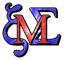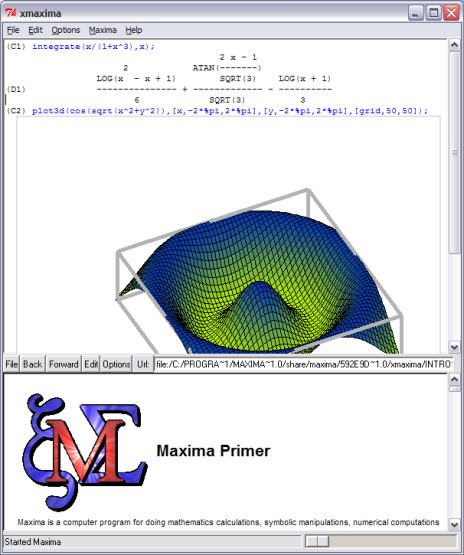Maxima is a system for the manipulation of symbolic and numerical expressions, including differentiation, integration, Taylor series, Laplace transforms, ordinary differential equations, systems of linear equations, polynomials, and sets, lists, vectors, matrices, and tensors. Maxima yields high precision numeric results by using exact fractions, arbitrary precision integers, and variable precision floating point numbers. Maxima can plot functions and data in two and three dimensions.

The Maxima source code can be compiled on many systems, including Windows, Linux, and MacOS X. The source code for all systems and precompiled binaries for Windows and Linux.

The constantly updating Maxima, to fixing bugs and improving the code and the documentation.

Maxima includes a complete programming language with ALGOL(ALGOrithmic Language)-like syntax but Lisp-like semantics. It is written in Common Lisp, and can be accessed programmatically and extended, as the underlying Lisp can be called from Maxima. It uses Gnuplot for drawing.